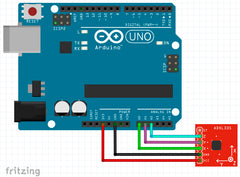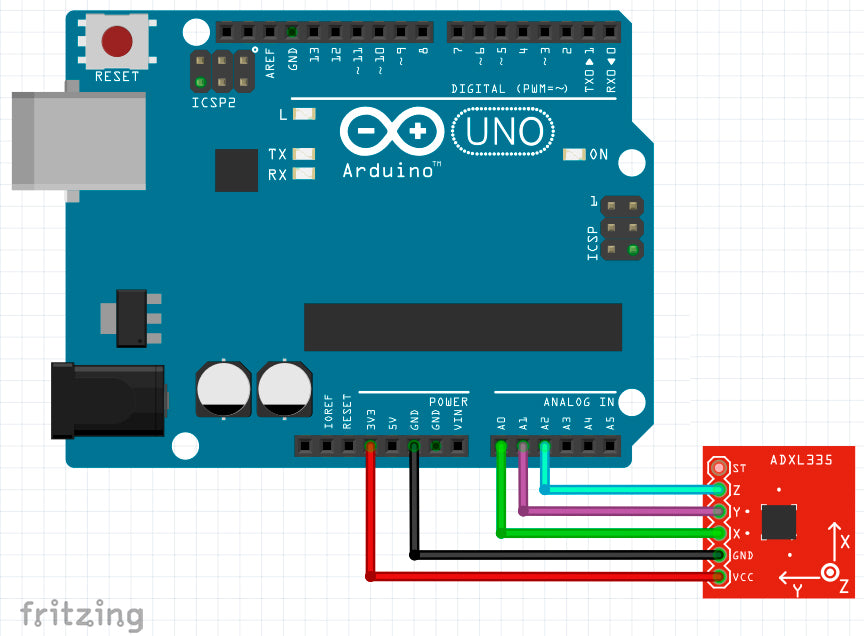# ➡️ How to connect analog accelerometr ADXL335 to Arduino

Instructions for connecting the accelerometer ADXL335 to the Arduino
We will need:
• Arduino UNO board or other compatible;
• connecting wires;

The principle of operation of the digital accelerometer

Accelerometers are used to determine the acceleration vector. The ADXL335 accelerometer has three axes, and due to this, it can determine the acceleration vector in three-dimensional space.

Due to the fact that the force of gravity is also a vector, we can determine the orientation of the accelerometer in three-dimensional space relative to the center of the Earth.

The illustration shows pictures from the passport for the ADXL335 accelerometer. It shows the coordinate axes of the sensitivity of the accelerometer with respect to the geometric placement of the device body in space, as well as acceleration values ​​are taken from the 3 channels of the accelerometer, depending on its orientation in space. The data are given for a sensor at rest, on which only the force of the earth acts.Let's take a closer look at what the accelerometer shows us.
Let the sensor lie horizontally, for example, on a table. Then the projection of the acceleration vector will be equal to "1g" along the Z axis, or Zout = 1g. The remaining two axes will be zeros: Xout = 0 and Yout = 0. When you rotate the sensor “on the back”, it will be directed in the opposite direction relative to the gravity vector, i.e. Zout = −1g. Similarly, measurements are taken on all three axes. It is clear that the accelerometer can be located as desired in space, so we will take non-zero readings from all three channels.

If the sensor is strongly shaken along the vertical Z axis, the Zout value will be greater than "1g". The maximum measured acceleration is “± 3g” on each of the axes (“plus” and “minus” here indicate the direction of acceleration).

I think, with the principle of operation of the accelerometer sorted out. Now consider the wiring diagram.

Connection diagram of the accelerometer to the Arduino

The ADXL335 analog accelerometer chip is rather small and placed in a BGA package, and at home, it is difficult to mount it on a board. Therefore, I will use a ready-made GY-61 module with an ADXL335 accelerometer.

To power the accelerometer, it is necessary to apply a voltage of +3.3 V to the VCC module output. The measuring channels of the sensor are connected to the analog outputs of the Arduino, for example, "A0", "A1" and "A2".Arduino has a 10-bit ADC, and the maximum allowable voltage at the output is 5 volts. The measured voltages are encoded with bits that can take on only 2 values ​​— 0 or 1. This means that the entire measurement range will be divided by (1 + 1) 10, i.e. on 1024 equal pieces. In order to translate the readings taken in volts, you need to divide each value measured at the analog input by 1024 (segment), and then multiply by 5 (volt).

Let's load this sketch into the Arduino memory. We will read indications from three channels from analog inputs, convert them to voltage and output them to the serial port.

```const int xPin = A0;
const int yPin = A1;
const int zPin = A2;

void setup() {
Serial.begin(9600);
}

void loop() {

Serial.print("x: ");
Serial.print(" | y: ");
Serial.print(" | z: ");

delay(100);
}```

Let's see what really comes from the accelerometer on the example of the Z axis (see the last column in the illustration). When the sensor is horizontal and looks up, the numbers come (2.03 ± 0.01). This should correspond to the acceleration "1g" along the Z axis and an angle of 0 ° according to the passport on the ADXL335. Let's reverse the sensor. The numbers come (1.69 ± 0.01), which should correspond to “−1g” and an angle of 180 °.Determination of accelerations in the three axes of the accelerometer

We remove the values from the accelerometer at angles of 90 ° and 270 ° and enter in the table. The table shows the angles of rotation of the accelerometer (column "A") and the corresponding Zout values in volts (column "B").For clarity, a graph of the voltage at the output Zout, depending on the angle of rotation. The blue field is a region of values ​​at rest (with the acceleration of 1g). The pink field on the graph is a margin so that we can measure acceleration up to + 3g and up to −3g.

At a rotation angle of 90 °, the Z-axis accounts for zero acceleration. Those. the value of 1.67 volts is the conditional zero Z0. Then we define the acceleration as follows:
g = Zout - Z0 / Sz,
here Zout is the measured value in millivolts, Z0 is the value at zero acceleration in millivolts, Sz is the sensor sensitivity along the Z axis, measured in mV / g.

Accelerometer sensitivity is given in the passport and is on average 300 mV / g or 0.3 V / g, but in general it is better to calibrate the accelerometer and calculate the sensitivity value specifically for your sensor using the formula:
Sz = Z (0 °) - Z (90 °)
In this case, the accelerometer sensitivity on the Z axis = 2.03 - 1.68 = 0.35 V / g. Similarly, the sensitivity will need to be calculated for the X and Y axes.

In the "C" column of the table, the calculated acceleration is given at a sensitivity equal to 350 mV / g. As you can see, the calculations almost coincide with the nominal values, which are given in the first figure from the passport to the ADXL335 sensor, i.e. Our sensor rather accurately shows its orientation in space (I showed it simply for self-testing, it will not be useful further).

Determination of the rotation angle of the accelerometer

Remembering the basic course of school geometry, we derive a formula for calculating the rotation angles of the accelerometer:
angle_X = arctan [√ (Gz2 + Gy2) / Gx].
Values are obtained in radians. To convert radians to degrees, divide the result by the number π and multiply by 180 °.

As a result, a full sketch that calculates the accelerations and angles of rotation of the accelerometer along all axes is shown in the sidebar. The comments provide explanations for the program code.

```const int xPin = A0;
const int yPin = A1;
const int zPin = A2;

const float Vmax = 5.0;

const float x0 = 1.71;
const float y0 = 1.69;
const float z0 = 1.68;

const float sens_x = 0.35;
const float sens_y = 0.35;
const float sens_z = 0.35;

void setup() {
Serial.begin(9600);
}

void loop() {

float Gx=(value_x*Vmax/1024.0 − x0)/sens_x;
float Gy=(value_y*Vmax/1024.0 − y0)/sens_y;
float Gz=(value_z*Vmax/1024.0 − z0)/sens_z;

Serial.print("Gx:\t" + String(Gx));
Serial.print("\t| Gy:\t" + String(Gy));
Serial.println("\t| Gz:\t" + String(Gz));

float angle_x = atan(sqrt(Gz*Gz + Gy*Gy) / Gx)*180 / PI;
float angle_y = atan(sqrt(Gx*Gx + Gz*Gz) / Gy)*180 / PI;
float angle_z = atan(sqrt(Gx*Gx + Gy*Gy) / Gz)*180 / PI;

Serial.print("x:\t" + String(round(angle_x)));
Serial.print("o\t| y:\t" + String(round(angle_y)));
Serial.println("o\t| z:\t" + String(round(angle_z)) + "o");
Serial.println();

delay(500);
}```

When outputting to the Serial.print () port, the \ t symbol denotes a tab character so that the columns are even and the values are located under each other. The + symbol means concatenation (union) of several strings. The String () operator explicitly tells the compiler to convert a numeric value to a string. The round () operator rounds the angle to within 1 °.So, we have learned how to capture and process data from the analog accelerometer ADXL335 using Arduino.